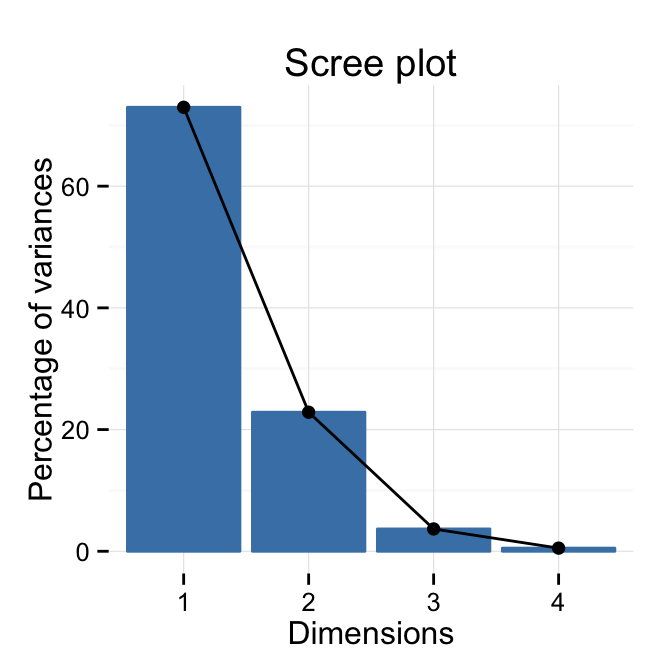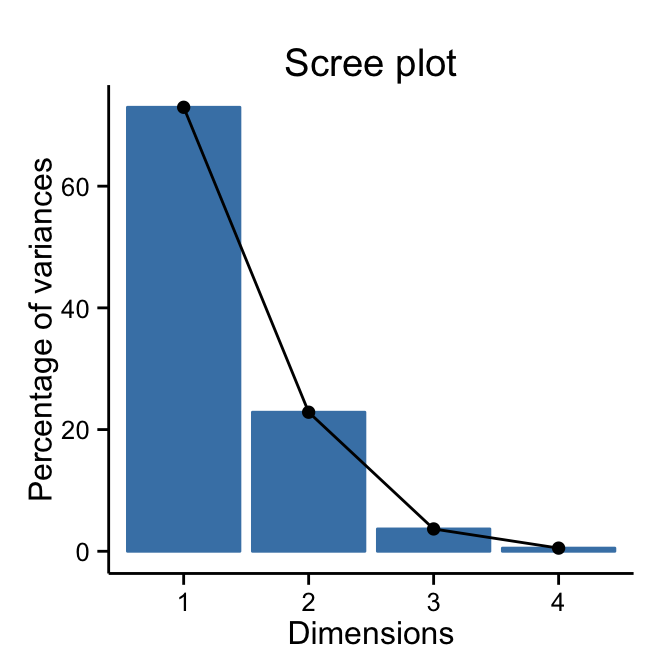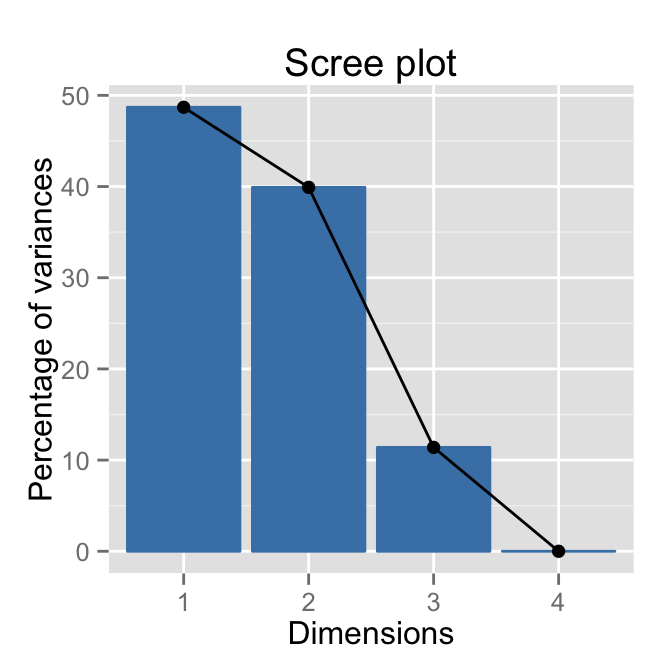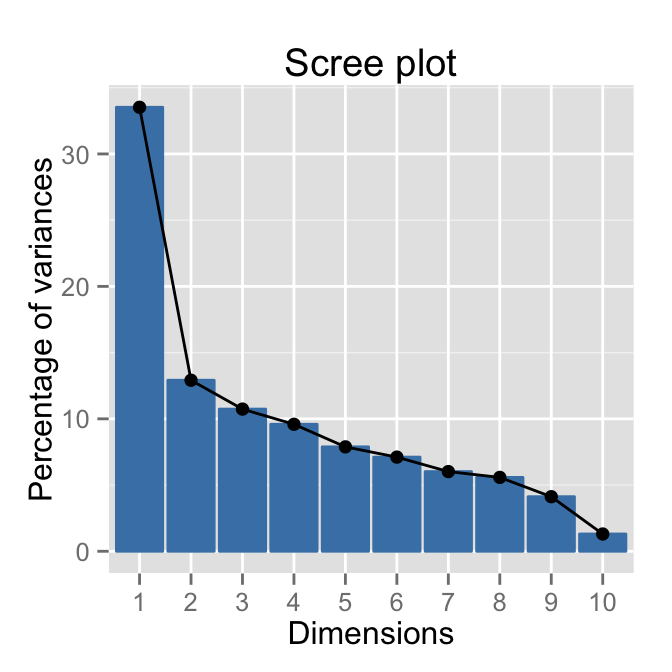# Description

This article describes how to extract and visualize the eigenvalues/variances of the dimensions from the results of Principal Component Analysis (PCA), Correspondence Analysis (CA) and Multiple Correspondence Analysis (MCA) functions.

The R software and factoextra package are used. The functions described here are:

• get_eig() (or get_eigenvalue()): Extract the eigenvalues/variances of the principal dimensions
• fviz_eig() (or fviz_screeplot()): Plot the eigenvalues/variances against the number of dimensions

The package devtools is required for the installation as factoextra is hosted on github.

``````# install.packages("devtools")
library("devtools")
install_github("kassambara/factoextra")``````

``library("factoextra")``

# Usage

``````# Extraction of the eigenvalues/variances
get_eig(X)
# Visualization of the eigenvalues/variances
fviz_eig(X, choice = c("variance", "eigenvalue"),
geom = c("bar", "line"), barfill = "steelblue",
barcolor = "steelblue", linecolor = "black",
ncp = 5, addlabels = FALSE, ...)
# Alias of get_eig()
get_eigenvalue(X)
# Alias of fviz_eig()
fviz_screeplot(...)``````

# Arguments

Argument Description
X an object of class PCA, CA and MCA [FactoMineR]; prcomp and princomp [stats]; dudi, pca, coa and acm [ade4]; ca and mjca [ca package].
choice a text specifying the data to be plotted. Allowed values are “variance” or “eigenvalue”.
geom a text specifying the geometry to be used for the graph. Allowed values are “bar” for barplot, “line” for lineplot or c(“bar”, “line”) to use both types.
barfill fill color for bar plot.
barcolor outline color for bar plot.
linecolor color for line plot (when geom contains “line”).
ncp a numeric value specifying the number of dimensions to be shown.
addlabels logical value. If TRUE, labels are added at the top of bars or points showing the information retained by each dimension.
optional arguments to be passed to the functions geom_bar(), geom_line(), geom_text() or fviz_eig().

# Value

• get_eig() (or get_eigenvalue()): returns a data.frame containing 3 columns: the eigenvalues, the percentage of variance and the cumulative percentage of variance retained by each dimension.
• fviz_eig() (or fviz_screeplot()): returns a ggplot2 plot.

# Examples

## Principal Component Analysis

A Principal Component Analysis (PCA) is performed using the built-in R function prcomp() and iris data:

``````data(iris)
``````  Sepal.Length Sepal.Width Petal.Length Petal.Width Species
1          5.1         3.5          1.4         0.2  setosa
2          4.9         3.0          1.4         0.2  setosa
3          4.7         3.2          1.3         0.2  setosa
4          4.6         3.1          1.5         0.2  setosa
5          5.0         3.6          1.4         0.2  setosa
6          5.4         3.9          1.7         0.4  setosa``````
``````# The variable Species (index = 5) is removed
# before the PCA analysis
res.pca <- prcomp(iris[, -5],  scale = TRUE)
# Extract the  eigenvalues/variances
get_eig(res.pca)``````
``````      eigenvalue variance.percent cumulative.variance.percent
Dim.1 2.91849782       72.9624454                    72.96245
Dim.2 0.91403047       22.8507618                    95.81321
Dim.3 0.14675688        3.6689219                    99.48213
Dim.4 0.02071484        0.5178709                   100.00000``````

## Visualize the eigenvalues/variances of the dimensions

``````# Default plot
fviz_eig(res.pca)````````````# Add labels``````# Change the y axis limits
fviz_eig(res.pca, addlabels=TRUE, hjust = -0.3) +
ylim(0, 80)````````````# Scree plot - Eigenvalues
fviz_eig(res.pca, choice = "eigenvalue",``````# Use only barplot``````# Use only lineplot
fviz_eig(res.pca, geom="line")````````````# Change theme
fviz_eig(res.pca) + theme_minimal()````````````# theme_classic()
fviz_eig(res.pca) + theme_classic()````````````# Customized plot
linecolor ="red") + theme_minimal()````````````# Change colors, y axis limits and theme
p <- fviz_eig(res.pca, addlabels=TRUE, hjust = -0.3,
barfill="white", barcolor ="darkblue",
linecolor ="red") + ylim(0, 85) +
theme_minimal()
print(p)````````````# Change titles
p + labs(title = "Variances - PCA",
x = "Principal Components", y = "% of variances")``````The following themes are available: theme_gray(), theme_bw(), theme_linedraw(), theme_light(), theme_minimal(), theme_classic().

## Correspondence Analysis

The function CA() in FactoMineR package is used:

``````library(FactoMineR)
res.ca <- CA(housetasks, graph = FALSE)
get_eig(res.ca)``````
``````        eigenvalue variance.percent cumulative.variance.percent
Dim.1 5.428893e-01     4.869222e+01                    48.69222
Dim.2 4.450028e-01     3.991269e+01                    88.60491
Dim.3 1.270484e-01     1.139509e+01                   100.00000
Dim.4 5.119700e-33     4.591904e-31                   100.00000``````
``fviz_eig(res.ca)``## Multiple Correspondence Analysis

The function MCA() in FactoMineR package is used:

``````library(FactoMineR)
data(poison)
res.mca <- MCA(poison, quanti.sup = 1:2,
quali.sup = 3:4, graph=FALSE)
get_eig(res.mca)``````
``````       eigenvalue variance.percent cumulative.variance.percent
Dim.1  0.33523140        33.523140                    33.52314
Dim.2  0.12913979        12.913979                    46.43712
Dim.3  0.10734849        10.734849                    57.17197
Dim.4  0.09587950         9.587950                    66.75992
Dim.5  0.07883277         7.883277                    74.64319
Dim.6  0.07108981         7.108981                    81.75217
Dim.7  0.06016580         6.016580                    87.76876
Dim.8  0.05577301         5.577301                    93.34606
Dim.9  0.04120578         4.120578                    97.46663
Dim.10 0.01304158         1.304158                    98.77079
Dim.11 0.01229208         1.229208                   100.00000``````
``fviz_eig(res.mca, ncp = 10)``# Infos

This analysis has been performed using R software (ver. 3.1.2) and factoextra (ver. 1.0.2)